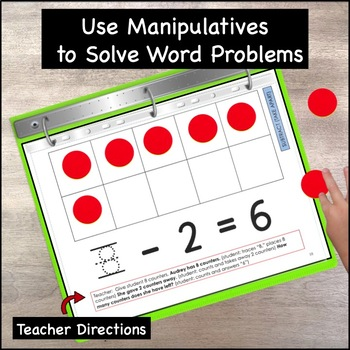# Basic Subtraction 0-10, Down Syndrome, autism, special educationSubject
Resource Type
Format
PDF (565 MB|156 pages)
Standards
\$18.00
\$18.00

#### Also included in

1. Designed especially for students with Down syndrome, autism, hearing and/ or visual impairment, and for students with intellectual disabilities.The Basic Addition and Subtraction Intervention Binders 0-10 can help if your math curriculum:•Moves too quickly for your students •Does not provide enough
\$32.40
\$36.00
Save \$3.60

### Description

Designed especially for students with Down syndrome, autism, hearing or visual impairment, and for students with intellectual disabilities.

The Basic Subtraction Intervention Binder 0-10

can help if your math curriculum:

•Moves too quickly for your students

•Does not provide enough repetition and practice before moving on

•Requires too much reading and writing

•Has too much vocabulary for students with delayed language skills

•Has numbers and pictures too small for your students to see easily

•Does not provide large enough spaces for children to write

•Presents too many problems on a page

What is the Basic Subtraction 0-10 Intervention Binder?

• It is a product for students who are struggling with the most basic concepts of subtraction. Each page contains only one problem.

• The binder includes a multitude of practice opportunities all in one convenient place. Struggling students can work in the binder with teachers, assistants, parents, and other professionals before moving on to higher concepts.

• It includes teacher/assistant instruction on every page including exact wordage to use with non-readers. Eliminates the problem of sharing one teacher guide when several adults are teaching. Each page also shows the concept taught in a blue tab, further simplifying the co-teaching and multiple-teacher model.

• Easy to use data sheets are provided to document progress.

• Strategies include using: pictures, ten-frames, counters, linking cubes, number lines, part, part whole mats, fingers, and drawing.

• An appendix includes templates for additional practice.

1) Subtract (Take From) 8

2) Minus Sign, Equal Sign 24

3) Subtract (Take Apart) 35

4) Model Subtraction Problems 47

5) Subtraction Sentences 58

6) Part-Part-Whole 74

7) Number Line to “Count Back” 95

8) Use Fingers to Subtract 111

9) Draw to Subtract 127

10) Appendix: Math Templates 138

Credits 156

There are 6 Ways to Use this Product:

Option 1: Print all pages on cardstock in color, laminate, hole punch and place in 3 ring binder.

Option 2: Print all pages on cardstock in grayscale, laminate, hole punch and place in 3 ring binder.

Option 3: Print in color OR black and white, place in page protectors, then in 3 ring binder.

Option 4: Choose one of the above methods, remove pages as needed so student can work on a flat or slanted surface.

Option 5: For pages using a ten-frame, place magnets on the bottom of 10 yellow and 10 red math counters. Have student use with page on dollar store sheet pan. (Usually becomes a favorite activity!)

Option 6: Print all or some pages on copy paper to use as worksheets.

What are the skills covered?

• Understand subtraction as taking apart and taking from

• Represent subtraction with objects, fingers, drawings, verbal explanations,
expressions, and equations

• Solve subtraction word problems

• Subtract within 10 by using objects or drawings to represent the problem

• Understand the meaning of the minus and equal signs

• In subtraction, number order matters

• When subtracting zero from a number, the result is the same number (Identity

• Use mental strategies such as “counting back”

• Decompose numbers less than or equal to 10 into pairs

• Subtraction within 10 using the part-part-whole model

• Use a number line as a tool for subtraction

MORE PRODUCTS THAT USE THE MATCH-SELECT-NAME METHOD:

Alphabet: Letter Recognition, Letter Identification, (Down Syndrome, spec. ed)

Total Pages
156 pages
N/A
Teaching Duration
N/A
Report this Resource to TpT
Reported resources will be reviewed by our team. Report this resource to let us know if this resource violates TpT’s content guidelines.

### Standards

to see state-specific standards (only available in the US).
Understand the meaning of the equal sign, and determine if equations involving addition and subtraction are true or false. For example, which of the following equations are true and which are false? 6 = 6, 7 = 8 - 1, 5 + 2 = 2 + 5, 4 + 1 = 5 + 2.
Add and subtract within 20, demonstrating fluency for addition and subtraction within 10. Use strategies such as counting on; making ten (e.g., 8 + 6 = 8 + 2 + 4 = 10 + 4 = 14); decomposing a number leading to a ten (e.g., 13 - 4 = 13 - 3 - 1 = 10 - 1 = 9); using the relationship between addition and subtraction (e.g., knowing that 8 + 4 = 12, one knows 12 - 8 = 4); and creating equivalent but easier or known sums (e.g., adding 6 + 7 by creating the known equivalent 6 + 6 + 1 = 12 + 1 = 13).
Understand subtraction as an unknown-addend problem. For example, subtract 10 – 8 by finding the number that makes 10 when added to 8.
Use addition and subtraction within 20 to solve word problems involving situations of adding to, taking from, putting together, taking apart, and comparing, with unknowns in all positions, e.g., by using objects, drawings, and equations with a symbol for the unknown number to represent the problem.
Solve addition and subtraction word problems, and add and subtract within 10, e.g., by using objects or drawings to represent the problem.